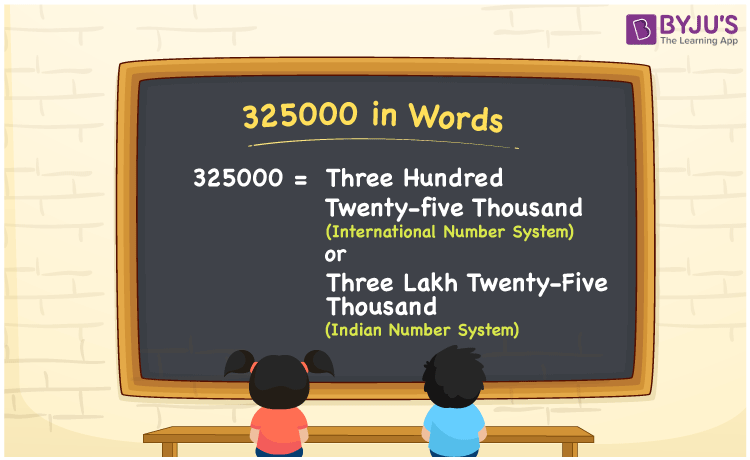# 325000 in Words

The number 325000 in words is written using both the Indian and the International Number System. In the Indian System of Numerals, the number 325000 in words is Three Lakh Twenty-Five Thousand. According to the International Number System, 325000 in words is Three Hundred Twenty-five Thousand. In this article, let us discuss how to write the number 325000 in words using the place value system with a complete explanation.

 325000 in Words (International Number System): Three Hundred Twenty-five Thousand 325000 in Words (Indian Number System): Three Lakh Twenty-Five Thousand. Three Lakh Twenty-Five Thousand in Numerical Form: 325000.

## 325000 in English Words## How to Write 325000 in Words?

The following is the place value table for the number 325000:

 Lakhs Ten-thousands Thousands Hundreds Tens Ones 3 2 5 0 0 0

The expanded form of 325000 is as follows:

= 3 × Lakh + 2 × Ten thousand + 5 × Thousand + 0 × Hundred + 0 × Ten + 0 × One

= 3 × 100000 + 2 × 10000 + 5 × 1000 + 0 × 100 + 0 × 10 + 0 × 1

= 300000 + 20000 + 5000

= 325000

= Three Lakh Twenty-Five Thousand

Hence, 325000 in words is Three Lakh Twenty-Five Thousand.

325000 in words – Three Lakh Twenty-Five Thousand or Three hundred twenty-five thousand

Is 325000 an odd number? – No

Is 325000 an even number? – Yes

Is 325000 a perfect square number? – No

Is 325000 a perfect cube number? – No

Is 325000 a prime number? – No

Is 325000 a composite number? – Yes

## Frequently Asked Questions on 325000 in Words

### How to write 325000 in words according to the Indian Number System?

According to the Indian Number System, 325000 in words is three lakh twenty-five thousand.

### How to write 325000 in words according to the International Number System?

According to the International Number System, 325000 in words is three hundred twenty-five thousand.

### Is 325000 a prime number?

No, 325000 is not a prime number.